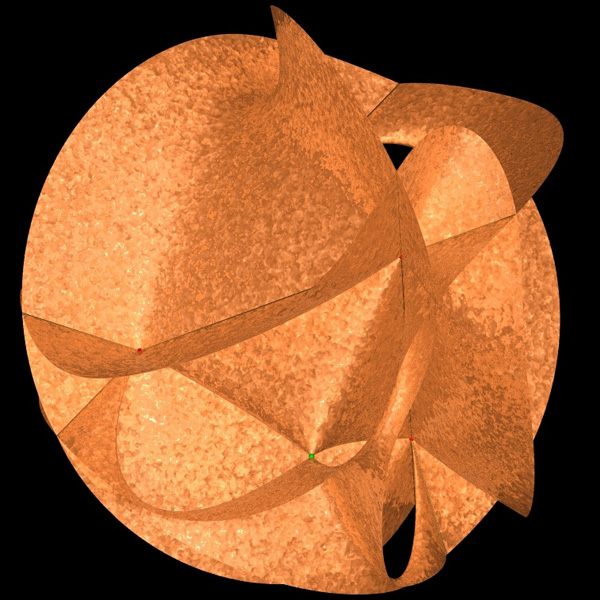# Sextic surfaces

Written by Paul Bourke
January 2006

Sextic equations are in general equations of a variable with power terms up to degree 6.

x6 + c5 x5 + c4 x4 + c3 x3 + c2 x2 + c1 x + c0 = 0

In 3 dimensions the equation contains all the possible terms in xn, yn, zn. In other words the sum over all the terms of the type shown below where m + n + o <= 6, there are 84 possible combinations so there are that many constants ci.

ci xm yn z oExample by Edmond Bonon, rendered in PovRay [sextic.inc]

Other examples of Sextic surfaces (degree 6) are the Barth Sextic, Hunt surface, and Boy surface.